# 深度学习 | 工具及实践（TensorFlow）

## TensorFlow编程框架、Graph、存储ckpt/PB、Eager Executon

Posted by JoselynZhao on May 5, 2019

# tensorFlow 基础：概念与编程模型

## TensorFlow 简介

• 开源的基于数据流图的数学计算库
• 多平台:支持 CPU/GPU，服务器、个 人电脑、移动设备
• 分布并行:方便多 GPU，分布式训练 可扩展:支持 OP 扩展，kernal 扩展
• 接口丰富:支持 python, java 以及 c++ 接口
• 可视化:使用 TensorBoard 可视化计 算图
• 易用性:相比 Caffe 等易于学习掌握， 文档资料丰富
• 社区支持:开源项目支持最多的几种 框架:Tensorflow、Caffe、pyTorch

## TensorFlow易于学习

• Python 接口:适于非计算机专业学习编程
• 安装方便: Anaconda 或 pip install
• 多平台:windows, linux, mac OS 模型设计方便:
1. 支持多种深度学习网络层
2. 自动求导，方便自定义层 (不用自己写求导公式)
3. 不用算每层的参数维度，自动计算
• 多 GPU, 分布式训练支持方便

## TensorFlow与python

Tensorflow(TF) 就是 Python 中调用的一个库

## Tensorflow 编程框架和机器学习模型对应关系

• 输入、输出、模型计算过程用计算图 Graph 描述
• 用优化器和训练数据对模型参数进行优化
• 模型设计围绕 Graph 展开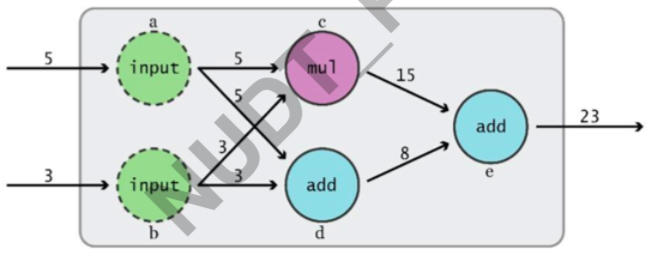## TensorFlow 基本原理

• TensorFlow=Tensor+flow 张量 (Tensor) 数据，流动 (flow) 的数据 Tensor 在哪里 flow?
• Graph: 计算图 Tensor 流动的路径图 Graphs 定义了模型和计算任务 Tensor 在图里怎么 flow 起来?
• Session: 会话 管理计算资源，驱动 Tensor 在 Graph 里 面转起来 怎么取数据?怎么取结果?怎么训练?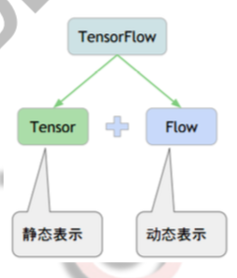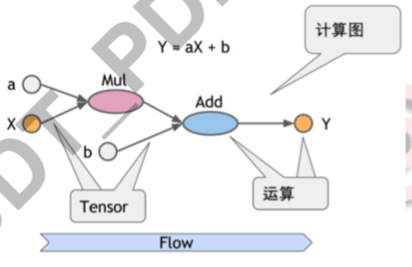## 编程模型

1. 画个图——定义模型计算图(Graph)
2. 写个执行剧本——定义会话(Session)，设计并执行计算过程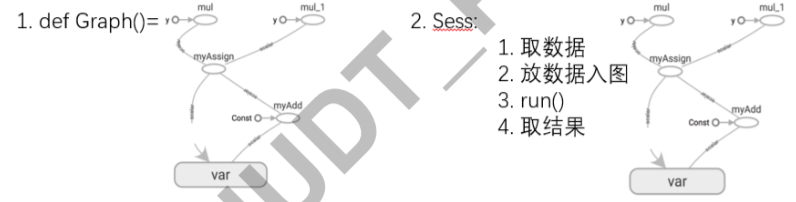## 计算图定义

Graph: 描述数学计算的有向图 (有向 无环图)

• 结构: 节点: 算子 (Operation):+-/… 连接节点的边:Operation 的输出或者 称作 (Tensor) 边缘点:(数据) 输入和 (参数) 变量
• 计算过程: 输入沿计算路径(有向)逐个结点激活 每个节点的激活需要所有前驱节点激活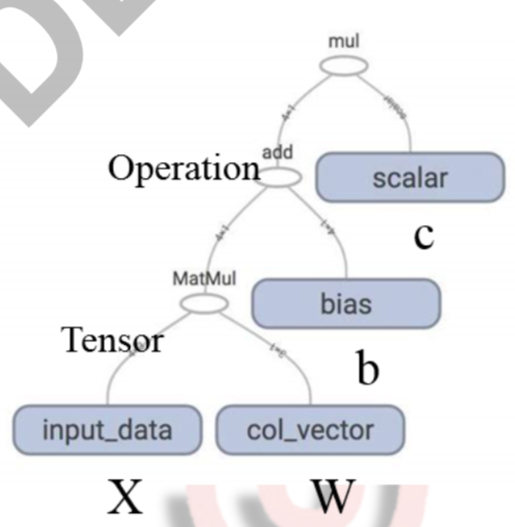上图为 $((w\times x)+b)\times c$的计算图

### Graph的边-张量

• 张量 (Tensor)——Graph 节点 (Operation) 之间传递的数据可以看作 n 维的数组 0 维张量: 标量(数) 1 维张量: 向量 2 维向量:矩阵 n 维向量……

• 张量 Tensor——算子 Operation 的输 出 引用中间计算结果

### 多维张量举例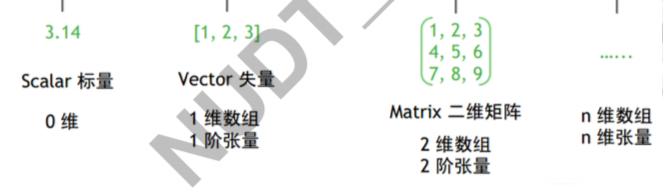### Graph 终端节点: 输入和模型参数

• tf.Variable: 变量节点 用来存储图执行过程中需要更新的量 在神经网络中用来存储权值
• tf.constant: 常量节点 在建立计算图时确定 要传入 python 值而非 tensor
• tf.placeholder: 占位节点 在运行时要给占位节点喂 (feed) 一个 值
• tf.zeros, tf.ones, tf.zeros_like, tf.ones_like, tf.random, …

### 计算流程与 Graph 的对应关

• 计算流程包括:算子、节点、数据传递
• 三个要素在 Graph 中的体现方式: 图中的节点:定义算子(Operation) 节点可以拥有零条或多条边:多输入，多输出 每条边表示节点的输入/输出，并以张量 (Tensor) 传递数据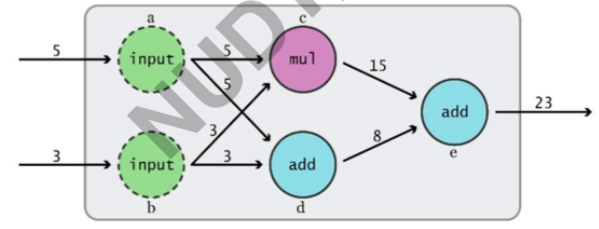### TF 中 Graph 构建方式

1. 像写函数一样，使用 tf 中的数据结构和算子 (tf.xxx) 直接描述 (整个描述成为模型默认图)
2. 可以指定多个图，并分别定义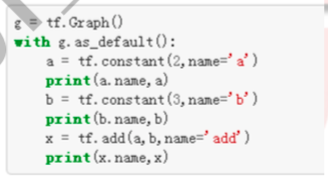### TensorFlow 静态图机制

Graph 构图与 python 函数计算区别:

1. Graph 的构建:使用 tf 中的数据结构和算子 (tf.xxx)，把整个模 型的所有连接都写一遍
2. Graph 构建的每个操作都要像函数一样执行一遍，否则无法建立 Graph
3. 构建的 Graph 仅仅定义了计算流程，不会给出计算结果 (Python 中则可以直接得到计算结果)
4. 要用 Graph 进行计算，需要在 Session 中给出输入和输出方式并 启动计算

### 静态图框架

• 代表:TensorFlow, Caffe
• 特点:预先定义计算图，运行时反复使用，不能改变
• 优点:速度更快，适合大规模部署，适合嵌入式平台
    import  tensorflow as tf
v1 = tf.Variable(tf.random_uniform())
v2 = tf.Variable(tf.random_uniform())
print(v1)
print(v2)
print(sum)

'''运行结果如下：
<tf.Variable 'Variable:0' shape=(3,) dtype=float32_ref>
<tf.Variable 'Variable_1:0' shape=(3,) dtype=float32_ref>


import tensorflow as tf

v1 = tf.Variable(tf.random_uniform())
v2 = tf.Variable(tf.random_uniform())

with tf.Session() as sess:
sess.run(tf.initialize_all_variables())
print(sess.run(v1))
print(sess.run(v2))
print(sess.run(sum2))

'''运行结果：
[0.6578543  0.625384   0.49183977]
[0.02306736 0.531626   0.8785937 ]
[0.6809217 1.15701   1.3704334]'''


### 动态图框架

• 代表:PyTorch
• 特点:每次运行时都会重新构建计算图，因此可以在学习过程中对计算图进行修改
• 优点:灵活性高，便于 debug，学习成本更低
 # 动态
import  torch
v1 = torch.rand(1,3)
v2 = torch.rand(1,3)
sum = v1+v2
print(v1)
print(v2)
print(sum)


## 运行模型-会话（Session）

• Session 用来给定 Graph 的输入，指定 Graph 中的结果获取方式， 并启动数据在 Graph 中的流动
• 拥有并管理 Tensorflow 程序运行时的所有资源
• 资源包括:硬件(CPU,GPU)，数据

• 默认调用 GPU:0
• 可以手动指定调用某个 GPU 或 CPU

    import tensorflow as tf
v1 = tf.constant([1.0,2.0,3.0],shape=,name='v1')
v2 = tf.constant([1.0,2.0,3.0],shape=,name='v2')
sum12 = v1+v2

with tf.Session(config=tf.ConfigProto(log_device_placement = True)) as sess:
print sess.run(sum12)

'''ConfigProto(log_device_placement = True) 的目的是为了在输出中指明cpu'''
'''运行结果如下：
[2. 4. 6.]
'''


    import tensorflow as tf

with tf.device('/CPU:0'):
v1 = tf.constant([1.0, 2.0, 3.0], shape=, name='v1')
v2 = tf.constant([1.0, 2.0, 3.0], shape=, name='v2')
sum12 = v1 + v2

with tf.Session(config=tf.ConfigProto(log_device_placement=True)) as sess:
print sess.run(sum12)


/cpu 或者/CPU 都是可以的

### Tensorflow 使用会话两种模式

1. 明确调用会话生成函数和关闭会话函数
2. 通过 Python 的上下文管理器来使用会话
	#会话模式1
sess = tf.Session()
sess.run()
sess.close()
#会话模式2
with tf.Session() as sess:
sess.run()


## TensorFlow 编程基本流程

    import tensorflow as tf
import numpy as np

a = tf.constant(1.,name='const1')
b = tf.constant(2.,name='const2')

with tf.Session() as sess:
print(sess.run(c))
print(c.eval)
# eval() 函数用来执行一个字符串表达式，并返回表达式的值。

'''运行结果如下：
3.0
<bound method Tensor.eval of <tf.Tensor 'Add:0' shape=() dtype=float32>>'''



## Numpy vs TensorFlow

numpy 实现：

    import  numpy as np
a  = np.random.rand(1)
for i in range(5):
print(a)

'''result:
[0.99835465]
[0.99835465]
[0.99835465]
[0.99835465]
[0.99835465]
'''
'''即生成的五个随机数是一样的'''


TensorFlow实现：

    import  tensorflow as tf
import  numpy as np

a = tf.random_normal(,name = "random")
with tf.Session() as sess:
for i in range(5):
print(sess.run(a))

'''result:
[-0.28919014]
[-0.516945]
[-0.5970153]
[1.6492158]
[0.2942117]'''
'''五个随机数各不相同'''


Numpy 到 TensorFlow 使用对应表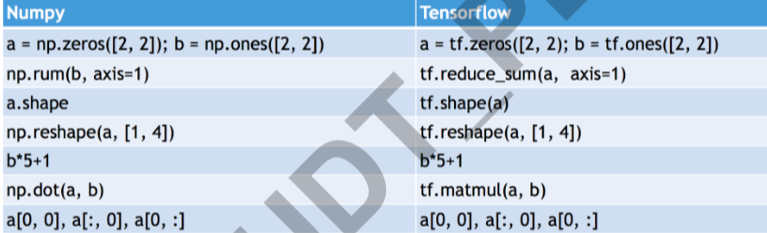## 小结

1. Tensorflow 是 Python 接口的深度学习计算库
2. TF 采用计算图描述模型，并用会话运行计算实例
3. TF 基本编程模式:计算图 + 会话

# TensorFlow 机器学习编程框架

## 使用 Scikit-learn 进行线性回归

    import sklearn
X_train,X_test,y_train,y_test = sklearn.model_selection.train_test_split(X,y,test_size = 0.2)
# 随机划分20%的数据作为测试集

clf = sklearn.linear_model.LinearRegression()
# 定义线性回归器

clf.fit(X_train,y_train) #开始训练
accuracy = clf.score(X_test,y_test) #测试并得到测试集性能


## tensorflow 和scikit-learn的区别

• TensorFlow 没有 Scikit-learn 当中预定义的各 种模型函数，如回归、神经网络等。
• 不能直接将数据以参数形式送入
• 必须手写计算图
• 计算图不参与计算
• Session 执行计算图

## Tensorflow 编程框架和机器学习模型的对应关系

• 输入、输出、模型计算过程用计算图 Graph 描述，并用优化器和训练数据对模型参数进行优化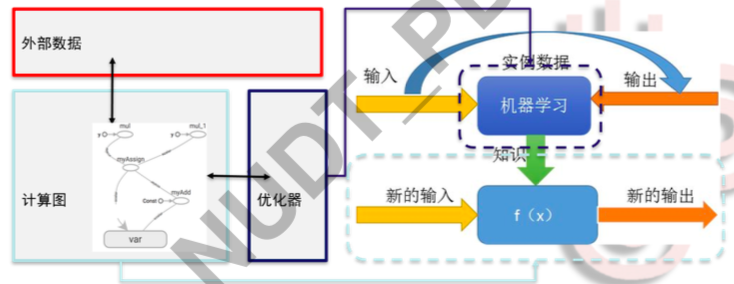• 机器学习模型 Graph= 计算路径+ 参数变量 采用静态图机制
• 优化 对象：参数变量 目标：损失函数最小 方法：梯度下降等

## TensorFlow的优化机制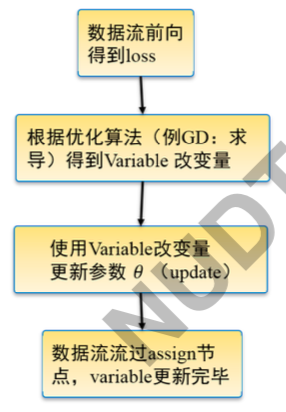## TensorFlow优化编程模式

1. 定义目标函数 (例: 损失函数 loss，模型预测与真值差距)
2. 基于目标函数和优化目标定义优化器 opt = tf.train.GradientDescentOptimizer(0.01) train_op = opt.minimize(loss)
3. 使模型获取数据并调用优化器进行训练 sess.run([train_op…],feed_dict=input_x:xxx, label:xx)

## TensorFlow机器学习基本编程框架

Graph建图

1. 创建数据，定义输入结点 (Placeholder)
2. 定义模型主要部分计算图 (Graph, Variable, …)
3. 定义损失函数 (loss)
4. 定义优化器及优化目标（train-op）

Session执行

1. 初始化参数 (initialization)
2. 定义(迭代)训练脚本并执行(fetch: train_op, feed: input_data, input_label)

## 1 创建数据，定义输入输出

feed 和 fetch 是 TensorFlow 模型与外界进行数据交互的方式

• 输入:Feed，将数据喂进 Tensorflow 实例图 (给 placeholder 节点)
• 输出:Fetch，Tensorflow 实例图中取数据 取哪个节点输出的值，就只计算计算图哪个部分 Fetch 一下，计算图就算一次，图里的 tensor 就更新一次
• 怎么喂?怎么拿? 输入:通过输入接口 a=tf.placeholder() 输出:指定 Graph 中的 tensor 作为输出具体形式:Sess.run ([c, …], feed_dict=a: xxxx, ….)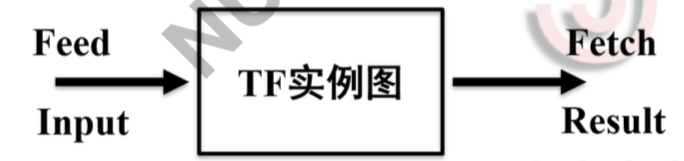• Placeholder = hold place: 占坑
• 仅仅起到占位符的作用
• 规定一些数据属性，但是不包括实际数据

• 启动会话将符合 placeholder 格式的数据送入计算图，并根据预先定义的计算方法进行运算
• 输出结果
• 手动 or 自动关闭会话

    import  tensorflow as tf
data = tf.placeholder(tf.float32,[None,4]) #后面是对输入数据规模的限制，4维
real_label = tf.placeholder(tf.float32,[None,1])
# 给定数据类型和数据大小。None表示本维度根据实际输入数据自适应调整


## 2 定义模型主要部分计算图

** 模型如何使用 Graph 定义：**

• 定义一组操作及操作附带的参数
• 例如:线性回归模型:(W * X) + b
• 参数在机器学习过程中不断被调整，一般用变量表示
• Graph:变量是依附其所属操作节点的 终端节点
• 变量为:W(权重)，b(偏置)。变量使用前要进行初始化

• 引用 tensor=tf.Variable(初始化值, 形状, 数据类型, 是否可训练? , 名 字, …)
• w=tf.Variable(initial_value=np.random.randint(10, size=(2,1)), name=’col_vector’, trainable=True)
• 变量初始化:参数初始化
• 形状, 数据类型暗含在初始化方法里

weight = tf.Variable(tf.random_normal([4, 1]), dtype=tf.float32)
bias = tf.Variable(tf.ones(), dtype=tf.float32) # 实际使用时只定义 了初值、变量规模和数据类型，默认可训练


## 3&4 定义损失函数、优化器和优化目标

y_label = tf.add(tf.matmul(data, weight), bias) # 定 义 回归函数的计算方法
loss = tf.reduce_mean(tf.square(real_label−y_label)) # 定义目标函数loss
train = tf.train.GradientDescentOptimizer(0.2).minimize (loss) # 定义优化器及优化目标(最小化loss), 其中0.2为 学习率


## 5 初始化参数

** 变量初始化的两个步骤:**

1. 定义变量时给定初始化值函数: a=tf.Variable(initial_value=…) b=tf.Variable(initial_value=…) …
2. Session 中执行初始化方法: … init= tf.global_variables_initializer() sess.run(init)

** 全局初始化与局部初始化:** 注意，即是用常量、随机数直接在变量定义时给定初始化值，变量 也此时也是没有值的，需要在 session 中运行初始化函数 全部初始化:tf.global_variables_initializer 部分初始化:tf.variables_initializer([a,b,…]) … init=tf.global_variables_initializer() sess.run(init)

with tf.Session() as sess: sess.run(tf.global_variables_initializer()) # 参 数

for i in range(1000): # 训 练1000次 迭 代 sess.run(train, feed_dict={data:X_train,
real_label:y_train}) # 执 行 训 练 脚 本
...


# TensorFlow线性回归

## 6 定义（迭代）训练脚本并执行

** 准备训练和测试数据:**

• X_train, X_test, y_train, y_test = sklearn.model_selection.train_test_split(X, y, test_size=0.2) # 随机划 分 20% 的数据作为测试集
• 有了训练数据和测试数据后，下面需要建立计算图

• data = tf.placeholder(tf.float32, [None, 4])
• real_label = tf.placeholder(tf.float32, [None, 1]) # 定义占位节点，数 据入口
• weight = tf.Variable(tf.random_normal([4, 1]), dtype=tf.float32)
• bias=tf.Variable(tf.ones(), dtype=tf.float32) # 定义参数节点

• y_label = tf.add(tf.matmul(data, weight), bias)
• loss = tf.reduce_mean(tf.square(real_label - y_label)) # 定义目标函数 loss
• train = tf.train.GradientDescentOptimizer(0.2).minimize(loss) # 定义优 化器及优化目标 (最小化 loss)

with tf.Session() as sess: sess.run(tf.global_variables_initializer()) # 参 数

for i in range(1000): # 训 练1000次 迭 代 sess.run(train, feed_dict={data:X_train,
real_label:y_train}) # 执 行 训 练 脚 本
forecast_set = sess.run(y_label, feed_dict={data: X_lately})# 执行测试。X_lately: 一部分不包括在 训练集和测试集中的数据，用于生成股价预测结果



    import  sklearn
import  tensorflow as tf

# 下面使用TensorFlow的方法

# ------------------准备训练和测试数据------------------------#
X_train, X_test, y_train, y_test = sklearn.model_selection.train_test_split(X, y, test_size=0.2)
# 随机划分20%的数据作为测试集

# ------------------placeholder------------------------#

data = tf.placeholder(tf.float32, [None, 4])
real_label = tf.placeholder(tf.float32, [None, 1])
# 给定数据类型和数据大小。None表示本维度根据实际输入数 据自适应调整

# -------------------定义变量-------------------#

weight = tf.Variable(tf.random_normal([4, 1]), dtype=tf.float32)
bias = tf.Variable(tf.ones(), dtype=tf.float32)  # 实际使用时只定义 了初值、变量规模和数据类型，默认可训练

# --------------------损失函数、优化器、优化目标----------------------#

y_label = tf.add(tf.matmul(data, weight), bias)  # 定 义 回归函数的计算方法
loss = tf.reduce_mean(tf.square(real_label - y_label))  # 定义目标函数loss
train = tf.train.GradientDescentOptimizer(0.2).minimize(loss)  # 定义优化器及优化目标(最小化loss), 其中0.2为 学习率

# ------------------初始化参数------------------------#
with tf.Session() as sess:
sess.run(tf.global_variables_initializer())
# 参 数初始化
for i in range(1000):  # 训练1000次迭代
sess.run(train, feed_dict={data: X_train, real_label: y_train})  # 执行训练脚本

# ------------------配置输入输出及优化器，并启动训练------------------------#
forecast_set = sess.run(y_label, feed_dict={data: X_test})
# 执行测试。X_lately: 一部分不包括在 训练集和测试集中的数据，用于生成股价预测结果

accuracy = tf.reduce_mean(tf.square(forecast_set - y_test))


## TensorFlow模型存储

1. Graph 结构
2. 变量值

1. ckpt 模式:
1. 计算图和变量分开保存
2. 读取模型时需要重新定义计算图，无需指明变量名
2. pb 模式: 封装存储方案，隐藏模型结构
1. 计算图和变量封装在一个文件中
2. 无需重新定义计算图，但是需要指出变量名

### ckpt模式

1. Meta graph: .meta 文件 protocol buffer 保存 graph. 例如 variables, operations, collections 等
2. Checkpoint file: .ckpt 文件 2 个二进制文件:包含所有的 weights, biases, gradients 和其他variables 的值。 mymodel.data-00000-of-00001 训练的变量值 mymodel.index
3. ’checkpoint’ 简单保存最近一次保存 checkpoint 文件的记录

Saver=tf.train.Saver(max_to_keep = 4,keep_checkpoint_every_n_hours = 2)
Saver.save(sess, ckpt_file_path, global_step)


saver.restore(sess,tf.train.latest_checkpoint(’./ckpt’))


### ckpt 模式存储选项

saver.save(sess, ’my_test_model’, global_step = 1000


saver.save(sess,’my_test_model’,global_step=1000,write_meta_graph=False)


saver = tf.train.Saver(max_to_keep=4, keep_checkpoint_every_n_hours=2)


	import tensorflow as tf

x = tf.Variable(tf.random_uniform())
y = tf.Variable(tf.random_uniform())
saver = tf.train.Saver()
with tf.Session() as sess:
sess.run(tf.global_variables_initializer())
print(sess.run(x))
print(sess.run(y))
print(sess.run(z))
save_path = saver.save(sess,save_file)

'''运行结果：
[0.6390506  0.26704168 0.09797013]
[0.98880136 0.55906487 0.00470507]
[1.627852   0.82610655 0.1026752 ]
并在save目录下参数相应的文件'''


1. 重复定义计算图为默认图，用 tf.train.Saver() 中的 restore 工具恢 复默认图
2. 指定.meta 文件中的计算图为所需恢复图，用该图的 Saver() 恢 复 获取图中张量:get_tensor_by_name(“name”) 需要记住图中张量的名字

 	import tensorflow as tf

x = tf.Variable(tf.random_uniform())
y = tf.Variable(tf.random_uniform())
saver = tf.train.Saver()
with tf.Session() as sess:
saver.restore(sess, save_file)
print(sess.run(x))
print(sess.run(y))
print(sess.run(z))

'''运行结果：
[0.6390506  0.26704168 0.09797013]
[0.98880136 0.55906487 0.00470507]
[1.627852   0.82610655 0.1026752 ]
与刚才存储的结果完全一样'''


### PB模式

PB 文件定义: MetaGraph 的 protocol buffer 格式的文件，包括计算图，数据流， 以及相关的变量等

PB 文件优点: 具有语言独立性，可独立运行，任何语言都可以解析 允许其他语言和深度学习框架读取、继续训练和迁移 TensorFlow 的模型 保存为 PB 文件时候，模型的变量都会变成常量，使得模型的大小 减小 可以把多个计算图保存到一个 PB 文件中 支持计算图的功能和使用设备命名区分多个计算图，例如 serving or training，CPU or GPU。

PB存储代码示例：

import tensorflow as tf
from tensorflow.python.framework import graph_util
x = tf.Variable(tf.random_uniform())
y = tf.Variable(tf.random_uniform())
with tf.Session() as sess:
sess.run(tf.global_variables_initializer())
print(sess.run(x))
print(sess.run(y))
print(sess.run(z))
constant_graph = graph_util.convert_variables_to_constants(sess, sess.graph_def, ['op_to_store'])
with tf.gfile.FastGFile(save_file, mode='wb') as f:
f.write(constant_graph.SerializeToString())

'''[0.5625318  0.71519125 0.34229362]
[0.49225044 0.16457498 0.53800344]
[1.0547823  0.8797662  0.88029706]
Converted 2 variables to const ops.'''


PB恢复代码示例：

import tensorflow as tf
from tensorflow.python.platform import gfile
# ...... something disappeared ......

with tf.Session() as sess:
with gfile.FastGFile(save_file, 'rb') as f:
graph_def = tf.GraphDef()
sess.graph.as_default()
tf.import_graph_def(graph_def, name='')
sess.run(tf.global_variables_initializer())
z = sess.graph.get_tensor_by_name('op_to_store:0') # x? y?
print(sess.run(z))

'''[1.0547823  0.8797662  0.88029706]'''
'''只取出了z的值'''


# Eager Execution

## TensorFlow 的调试问题

• TensorFlow 程序的输入采用 placeholder 模式，难以指定具体输入 数据进行调试
• Session.run() 的运行模式降低了调试效率
import tensorflow as tf
import numpy as np
x = tf.placeholder(tf.float32, [None, 1])
m = tf.matmul(x, x)
with tf.Session() as sess:
sess.run(tf.global_variables_initializer())
m_out = sess.run(m, feed_dict={x: [[2.]]})
print(m_out) #运行结果如下: '''
[[4.]]
'''


## TensorFlow placeholder 模式的调试问题

import tensorflow as tf
x = [[2.]]
m = tf.matmul(x, x)
print(m)
#运行结果如下:
'''
Tensor("MatMul:0", shape=(1, 1), dtype=float32) '''


## Eager Execution

• TensorFlow: 静态图机制
• PyTorch: 动态图机制，调试更加灵活
• Google Brain 团队于 2017 年年底发布 Eager Execution 机制: ” 今天，我们为 TensorFlow 引入了「Eager Execution」，它是一个命 令式、由运行定义的接口，一旦从 Python 被调用，其操作立即被执 行。这使得入门 TensorFlow 变的更简单，也使研发更直观。”

### Eager Execution 的优势

• 快速调试即刻的运行错误并通过 Python 工具进行整合
• 借助易于使用的 Python 控制流支持动态模型
• 为自定义和高阶梯度提供强大支持
• 适用于几乎所有可用的 TensorFlow 运算

### Eager Execution 的使用

• Eager Execution 采用直接定义输入变量的模式，不使用 placeholder
• 当启动 Eager Execution 时，运算会即刻执行，无需 Session.run() 就 可以把它们的值返回到 Python
if __name__ =="__main__":
import  tensorflow as tf
import  tensorflow.contrib.eager as tfe
tfe.enable_eager_execution()
x = [[2.]]
m = tf.matmul(x,x)

print(m)
'''tf.Tensor([[4.]], shape=(1, 1), dtype=float32)'''


Eager Execution 中不能自动调用 GPU 资源 如果要在 Eager Execution 中使用 GPU 计算资源，则需要显式地将 tensor 移动到指定的 GPU 中

a = a.gpu() # copies tensor to default GPU (GPU0)
a = a.gpu(0) # copies tensor to GPU0
a = a.gpu(1) # copies tensor to GPU1
a = a.cpu() # copies tensor back to CPU


# 总结

• TensorFlow 基本概念和基本编程模型:计算图和会话
• 基本 TensorFlow 机器学习编程框架
• 模型存储的两种模式 ckpt 和 PB. 其中 ckpt 方便灵活，PB 模式适用 于模型封装和移植
• Eager Execution: TensorFlow 的动态图模式

# 源码